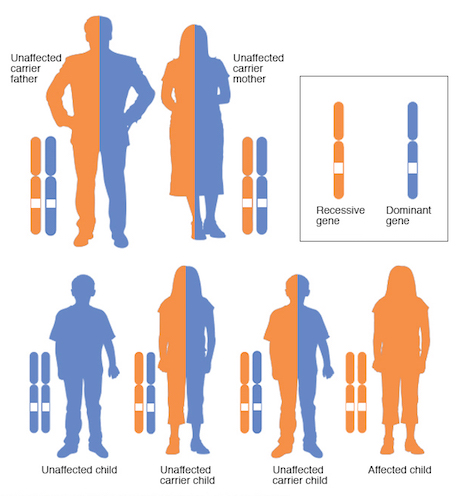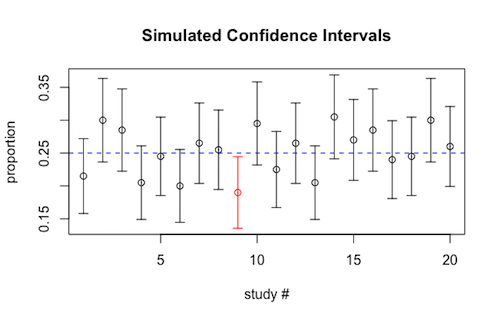## Modeling Statistical Confidence

To better understand confidence intervals, we would like to create a visualization of what "95% confidence" actually means.

To provide some context, let us consider the case of autosomal recessive inheritance of a genetic disorder, where two unaffected people who each carry one copy of the mutated gene for the disorder in question have a $0.25$ chance with each pregnancy of having a child affected by the disorder.Suppose a geneticist wants to verify this fact, and conducts a study (using mice), where 200 such pregnancies are examined, and the proportion of born mice with the disorder is found, and a 95% confidence interval for the probability one will have the disorder (which we already know to be $p=0.25$) is constructed.

We would like to simulate a study (or studies) similar to this one and their resulting confidence intervals in R. As such,

1. Write an R function simulated.sample.proportions(x,n,p) that simulates $x$ studies of $n$ pregnancies each with probability $p$ of the disorder, and returns the sample proportions found for each as a single vector.

2. Write an R function margin.of.error(p.hat,n,conf.level) that calculates the margin of error for a confidence interval for a proportion with the given confidence level (conf.level), sample proportion (p.hat), and sample size $n$.

3. Write an R function simulated.confidence.intervals(x,n,p,conf.level) that uses the previously created functions to simulate $x$ studies of $n$ pregnancies with probability $p$ of the disorder and creates a graph of the confidence intervals for $p$ each study produces (at the given confidence level). Use the plotCI() function of the plotrix package to produce the graph.

In the graph, draw a dashed horizontal line in blue at the height that corresponds to the proportion $p$. Also, color the confidence intervals that do not cross this horizontal line (and thus do not contain $p$) in red. Make sure your graph has an appropriate title and labels for the $x$ and $y$ axes.

Finally, your function should return the proportion of confidence intervals that contain $p$. A sample run of this function to simulate 20 studies similar to the one the aforementioned geneticist was said to have conducted above is provided below:

> simulated.confidence.intervals(20,200,0.25,0.95)
 0.954. Increase the number of studies from the 20 used in the sample run above to 50 and then run this function several times, varying the confidence level each time. Explain the differences you see -- especially as it relates to:

1. the proportion of intervals that capture the true probability $p$ that one will have the disorder (either from the graph or the value returned by the function), and

2. the width of the confidence intervals produced. (Note, you will want to pay attention to the scale of the $y$-axis, which may change from run to run.)

Did you expect the differences seen? Why?

5. Run this function several times, this time varying only the number of pregnancies examined in each study. Again, explain the differences you see.